## 65) Subtraction

Bonds of 11 to 20: Difference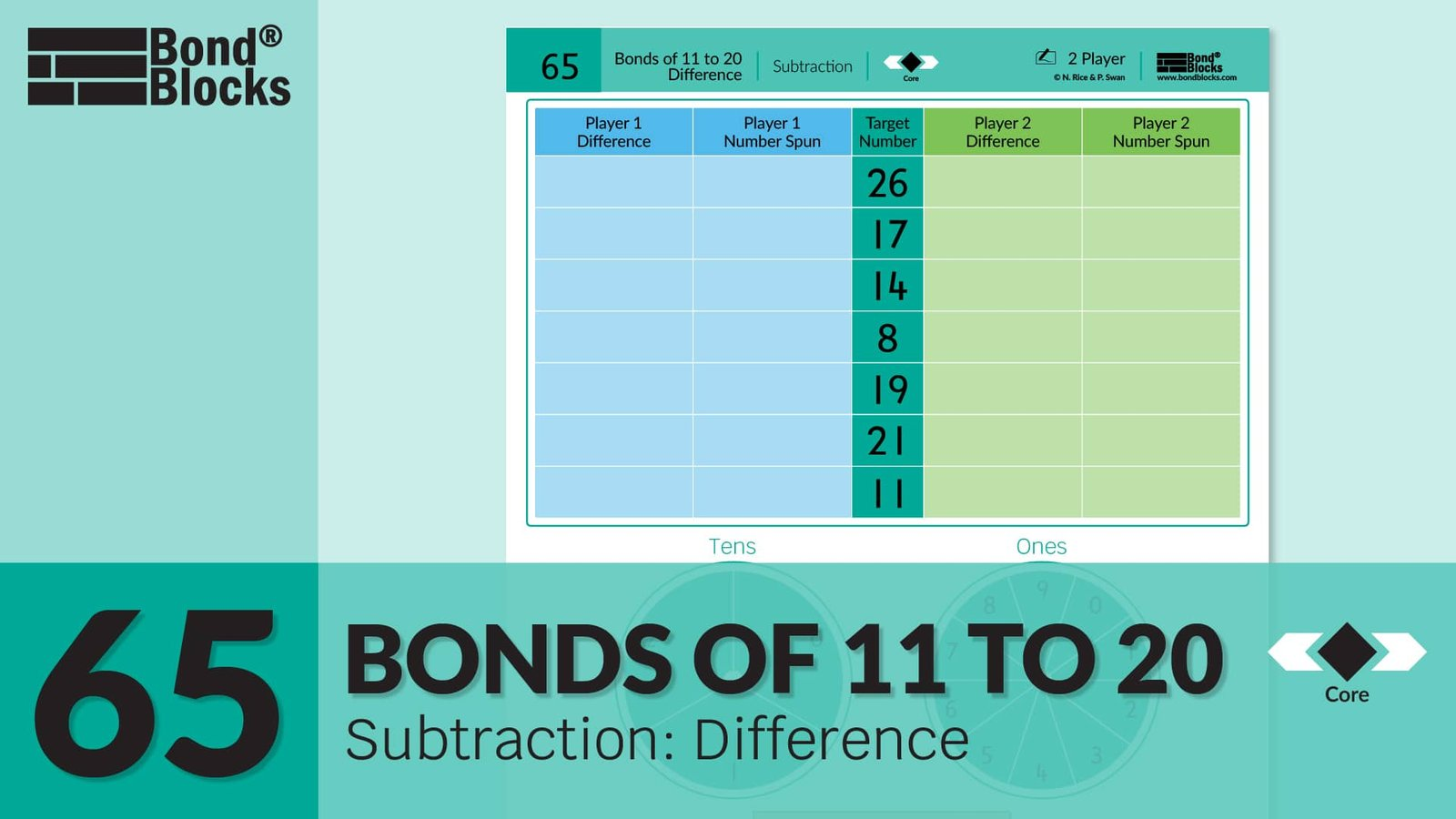### Mathematics

Develop fluency calculating the difference between two, two-digit numbers less than 30.

Use part-part-whole to develop the concept of finding an unknown part in a subtraction equation by either:

• Adding on to the known part to equal the whole or

Known Part + Unknown Part = Whole

• Subtracting known part from the whole.

Whole – Known Part = Unknown Part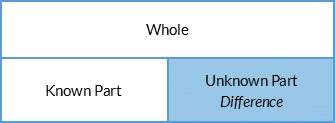In this activity the:

• Whole could be either the Target Number or the Number Spun.
• Known part could be either the Target Number or the Number Spun.
• Difference between these two numbers is the unknown part.

The player who flicked the spinner calculates the unknown part using adding on.
The other player checks the calculation of the unknown part using subtraction on a calculator.

For example, Target Number of 14, spin 9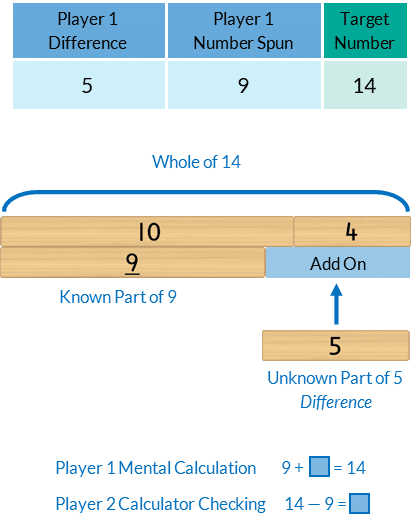For example, Target Number of 14, spin 21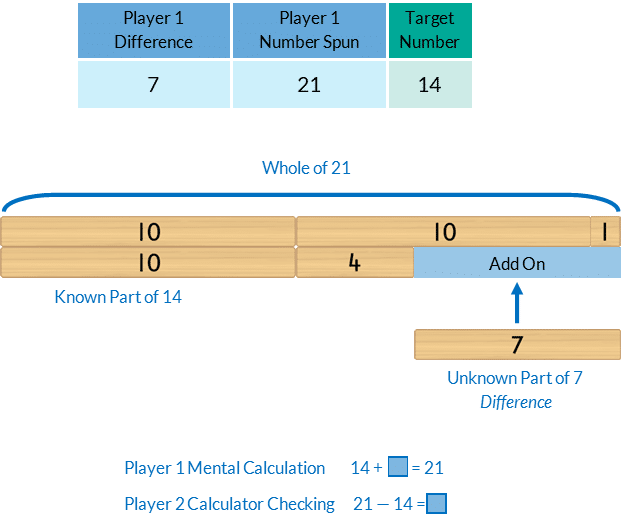Prior knowledge of part-part-whole, ten plus bonds and bridging ten is required.

### Language

• a number is made up of digits. For example, the number 23 is made up of two digits, 2 and 3.
• place value: The place of a digit in a number, in relation to the ones place, determines the digit’s value or worth. For example, In the number 23,
• the digit 2 is in the tens place, so is worth two tens, 20.
• the digit 3 is in the ones place so is worth three ones, 3.
• subtraction
• equals
• difference: the amount by which two numbers differ.## Differentiation

### A little easier

##### Use Bond Blocks

Use Bond Blocks to represent the whole, as well as the parts.Bond Blocks can be a helpful scaffold when bridging ten to calculate the unknown part. For example,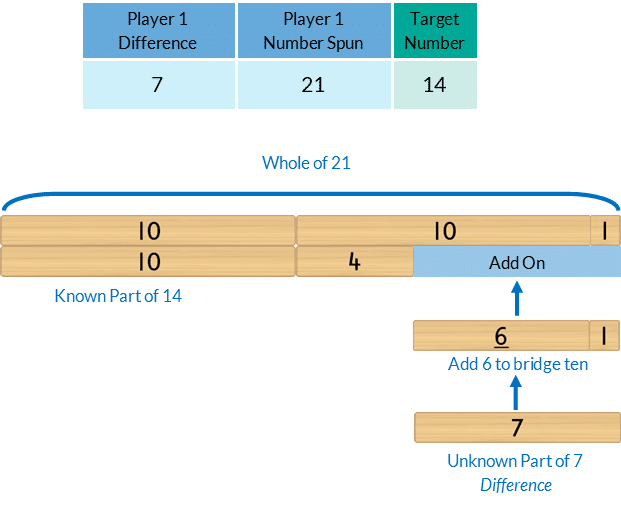### A little harder

##### Lowest overall difference

After the final round each player calculates their overall difference by adding all of their difference scores from each round, without using a calculator. The player with the overall lowest overall difference is the winner.  Bond Blocks can be used to support calculations where needed. Each player checks the other player’s total difference using a calculator.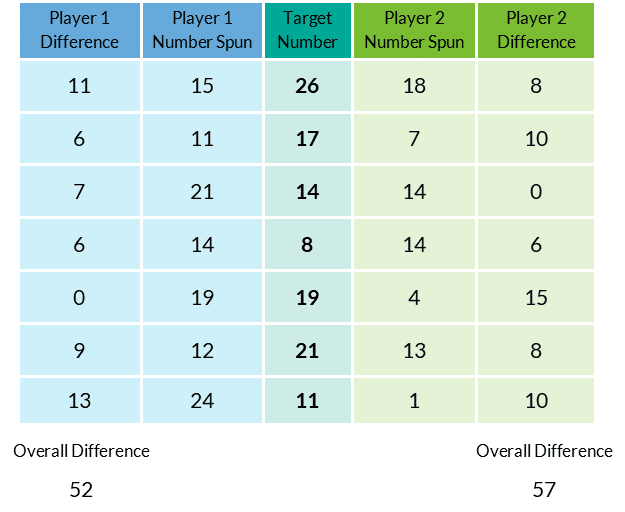Here is an example of how Bond Blocks could be used to support adding Player One’s difference scores.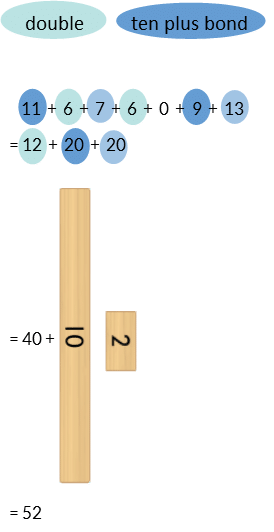Here is an example of how Bond Blocks could be used to support adding Player Two’s difference scores.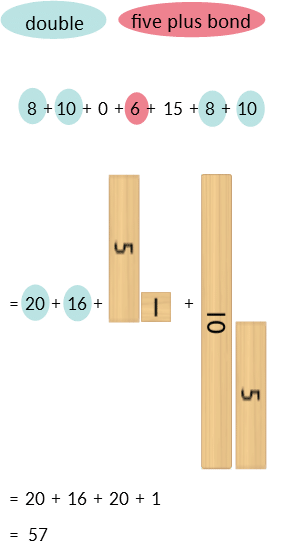### Progression

In the next activity, students use addition and subtraction to find missing wholes and parts. Go to

##### Activity 66

Bonds of 11 to 20: Equation, Building